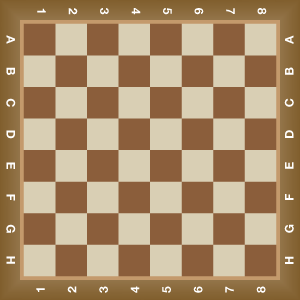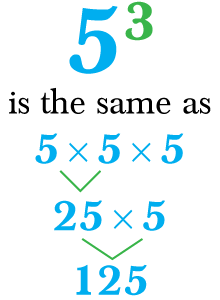# Exponents

Go back to  'Exponents, Squares, Cubes'

## Introduction to Exponents

Long years back, there was a King who also loved to play the game of chess. One day a travelling hermit visited his court. As was his habit, the King challenged him to a game of chess, and also said that if he won, he could choose his own reward. The hermit said that if he won, the King should give him as many grains of rice as could be placed on a chessboard in the following manner : one grain on the first square, two on the second, four on the fourth, eight on the fifth, and so on, doubling the number of grains at each square. The King laughed at this strange and humble sounding demand for (what he thought was) a handful of rice grains and began to play. To his misfortune, the King lost the game, and the measuring of the rice grains began. There are 64 squares in a chess board, and here are the rice grains for just the first 15 of them:Square 1:  1
Square 2:  2
Square 3:  4
Square 4:  8
.
.
.

Square 15:  16384

As you can see, just the first 15 squares out of the 64 gave the hermit a total of more than 32000 grains!! This is called the power of compounding, because in each step the number is doubled. Suffice to say that this seemingly small number of 2 multiplied 64 times ensured that the King had to give a huge quantity of rice grains to the hermit.

## The Big Idea: What are exponents?

Just like 2 was multiplied over and over again in the above example, exponents are the word used to explain the operation of multiplying a certain number by itself. So if you multiply the number 5 by itself seven times then you say that 7 is the exponent of the base 5, and this is how the number is written and calculated:

\begin{align}5 \times 5 \times 5 \times 5 \times 5 \times 5 \times 5 = {5^7} = 78125\end{align}

In the above example, 5 is the base, while 7 is the exponent and is also called power. So, 57 can be described as the base 5 raised to the power 7.

Two of the most commonly used exponents are the power of 2 and 3. When a base number is raised to the power 2 it is called a square, and when a base number is raised to the power 3, it is called a cube. So, 72 is the square of 7 and equal to 7 x 7 = 49, while 53 is the cube of 5, and is equal to 5 x 5 x 5 = 125.## Why exponents are important?

In the story of the King and hermit above, if the hermit was to tell the number of grains to the King, neither would he be able to explain or name the number properly, nor would the King be able to comprehend the number easily.

### The concept of negative exponents

Sometimes we see negative exponents used to describe numbers. To understand the use of negative exponents, we need to know what an inverse is. The inverse of any number is the number obtained by dividing 1 by that number. So, the inverse of 5 is $${1 \over 5}$$, and the inverse of 22 is  $${1 \over22}$$.

When you see a number with a negative exponent, it also means that the same number is divided by one. Here are two examples for you to understand:

\begin{align} &{5^{ - 2}} = \frac{1}{{{5^2}}} = \frac{1}{{25}}\\ & {x^{ - 8}} = \frac{1}{{{x^8}}} \end{align}

### Operations using exponents

The multiplication and division of numbers using exponents uses additions and subtraction. Let us see this with an example.
Say you wish to multiply 25 x 28 Instead of multiplying 25 with 28, you can write the expression as:

\begin{align} {2^5} \times {2^8} &= {2^{(5\, + \,8)}}\\ & = {2^{13}} = 8192 \end{align}

Similarly, when we need to divide, then we subtract.

\begin{align} \frac{{{3^8}}}{{{3^5}}} &= {3^{(8 - 5)}}\\ & = {3^3} = 27 \end{align}

You must remember that these rules of addition and subtraction can be applied only when the base is the same. In the first example, the base for both numbers to be multiplies was 2, which allowed us to add the exponents. Similarly, for the second example, the base for both numbers was 3, which allowed us to subtract the exponents.

Exponents